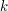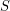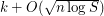Пятница 27.12. Дмитрий Соколов: "Trade-offs Between Size and Degree in Polynomial Calculus"

Пятница, 27 декабря, ауд. 106. Начало в 12:00.

Докладчик: Дмитрий Соколов.

Тема: Trade-offs Between Size and Degree in Polynomial Calculus.

Abstract

Building on [Clegg et al.~'96], [Impagliazzo et al.~'99] established that if an unsatisfiable-CNF formula over~variables has a refutation of size~in the polynomial calculus resolution proof system, then this formula also has a refutation of degree. The proof of this works by converting a small-size refutation into a small-degree one, but at the expense of increasing the proof size exponentially. This raises the question of whether it is possible to achieve both small size and small degree in the same refutation, or whether the exponential blow-up is inherent. Using and extending ideas from [Thapen~'16], who studied the analogous question for the resolution proof system, we prove that a strong size-degree trade-off is necessary.